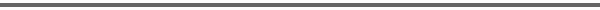Journal Home Page Cumulative Index List of all Volumes Complete Contentsof this Volume Previous Article Next Article Journal of Convex Analysis 11 (2004), No. 2, 363--385 Copyright Heldermann Verlag 2004Homogenization of Evolution Problems in a Fiber Reinforced Structure Michel Bellieud Dep. de Mathematiques, Universite de Perpignan, 52 av. de Villeneuve, 66100 Perpignan, France, bellieud@univ-tln.fr[Abstract-pdf] We study the homogenization of parabolic or hyperbolic equations like $$\rho_\epsilon(x){\partial^n u_\epsilon \over \partial t^n}- div(a_\epsilon(x) \nabla u_\epsilon) =f$$ on $\Omega\times (0, T)$ plus {\sl boundary conditions}, $n \in \{1,2\}$, where the coefficients $a_\epsilon$ and $\rho_\epsilon$ takes values of very different order on an $\epsilon$-periodic subset $T_\epsilon \subset \Omega$ (fibered structure) and elsewhere. We find a non local effective equation deduced from a homogenized system of several equations. Keywords: homogenization, fiber structures, two-scale convergence, Γ-convergence. MSC 2000: 35B40, 35J55, 74B05, 74K10, 74Q15. FullText-pdf (764 KB) for subscribers only.